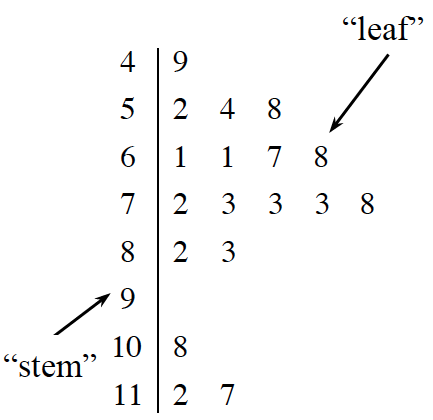### Home > ACC7 > Chapter 1 Unit 1 > Lesson CC3: 1.1.4 > Problem1-38

1-38.

Consider this data: $22$, $15$, $30$, $51$, $27$, $33$, $19$. Homework Help ✎

1. Arrange the data into a stem-and-leaf plot. (Refer to the glossary if you need a reminder of what a stem-and-leaf plot is.)

2. Find the mean and median.

3. If the value $51$ was replaced with $33$, which measure(s) of central tendency would change and which would not? Explain.

stem-and-leaf plot    A frequency distribution that arranges data so that all digits except the last digit in each piece of data are in the stem, the last digit of each piece of data are the leaves, and both stems and leaves are arranged in order from least to greatest.  The example at right displays the data:
$49, 52, 54, 58, 61, 61, 67, 68, 72, 73, 73, 73, 78, 82, 83, 108, 112,$ and $117$.mean   The mean, or average, of several numbers is one way of defining the “middle” of the numbers.  To find the average of a group of numbers, add the numbers together then divide by the number of numbers in the set. For example, the average of the numbers $1$, $5$, and $6$ is $(1+5+6)\div3=4$.  The mean is generally the best measure of central tendency when there are not outliers in the data set. See average.

median    The middle number of an ordered set of data.  If there is no distinct middle, then the average of the two middle numbers is the median.  The median is generally more accurate than the mean as a measure of central tendency when there are outliers in the data set.

measure of central tendency   Mean and median are measures of central reflecting special statistical information about a set of data.